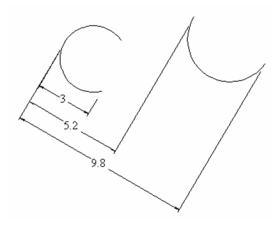# Quick Dimensions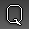Default UI Menu: Dimension/Quick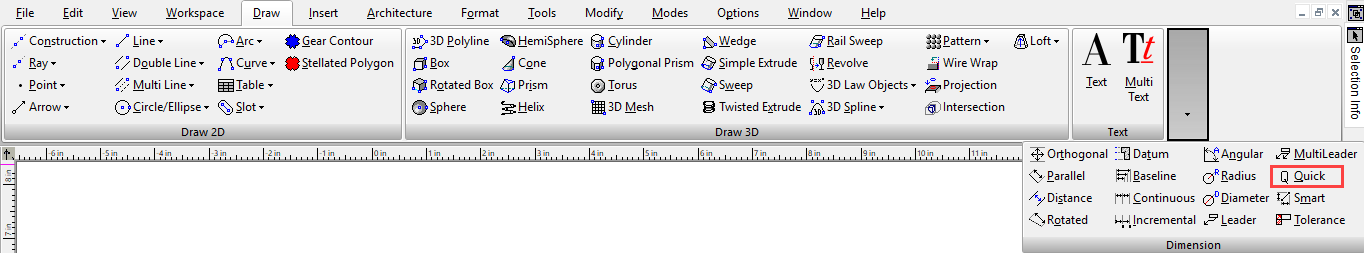Creates a series of dimensions on one more objects. You can choose the type of dimension and which points will be included.

Note: To create a group of various types of dimensions automatically,

Activate the Quick function, then choose three criteria: Whether the dimensions will be orthogonal (horizontal / vertical. The type of dimension (continuous, baseline, etc.), The points between which the dimensions will be created. In this example, the dimensions will be Orthogonal and Continuous (indicated by the arrows in the picture below). Points will be described later in the example.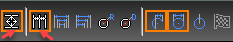Select the object or objects to dimension. You can use the Shift key to select multiple objects, or drag a selection window. This example consists of one circle and one polyline.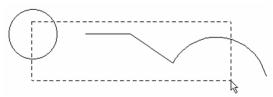Double-click when all objects are selected. Then move the cursor and click to place the dimensions. Moving the mouse to the right or left of the objects creates vertical dimensions; moving above or below the objects, as shown below, creates horizontal dimensions.This would be the result with Staggered dimensions:This would be the results with Baseline dimensions.If Radius is selected, a radius dimension is assigned to all arc or circle segments found in the selected objects.If Diameter is selected, a diameter dimension is assigned to all arc or circle segments found in the selected objects.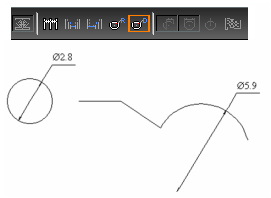Points affect dimensioning of arcs, curves, and segments of polylines. Start / End creates dimensions between all start and end points of arcs.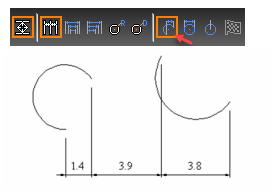Dimension measures the overall distance of the objects.Arc Center measures between center points.You can combine Points options. This example uses both Dimension and Arc Center.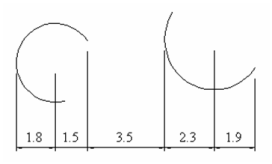If Orthogonal is not selected, you can define the dimension direction. Select two points to define the direction normal to the desired dimension line.This is the result of non-orthogonal, baseline dimensions.